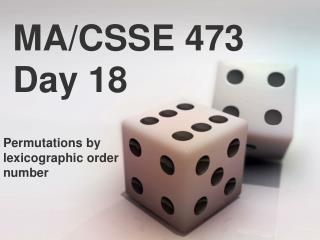Download PresentationMA/CSSE 473 Day 18# MA/CSSE 473 Day 18 - PowerPoint PPT Presentation

Download Presentation##### MA/CSSE 473 Day 18

Download Policy: Content on the Website is provided to you AS IS for your information and personal use and may not be sold / licensed / shared on other websites without getting consent from its author. While downloading, if for some reason you are not able to download a presentation, the publisher may have deleted the file from their server.

- - - - - - - - - - - - - - - - - - - - - - - - - - - E N D - - - - - - - - - - - - - - - - - - - - - - - - - - -
##### Presentation Transcript

1. MA/CSSE 473 Day 18 Permutations by lexicographic order number

2. MA/CSSE 473 Day 18 • Today's in-class quiz is a continuation of yesterday's • Tuesday will be another "ask questions prior to the exam" day. • Exam details are in previous PowerPoint slides. • Today's topics: • Permutations by lexicographic order number • Generating subsets of a set.

3. Horner's method code

4. Permutations and order • Given a permutation of 0, 1, …, n-1, can we directly find the next permutation in the lexicographic sequence? • Given a permutation of 0..n-1, can we determine its permutation sequence number? • Given n and i, can we directly generate the ith permutation of 0, …, n-1?

5. Yesterday's Discovery • Which permutation follows each of these in lexicographic order? • 183647520 471638520 • Try to write an algorithm for generating the next permutation, with only the current permutation as input.

6. Lexicographic Permutation class • These are the basics of the class setup. • The next() method provides an iterator over the permutations. How should it get from one permutation to the next?

7. Main permutation method

8. More discoveries • Which permutation follows each of these in lexicographic order? • 183647520 471638520 • Try to write an algorithm for generating the next permutation, with only the current permutation as input. • If the lexicographic permutations of the numbers [0, 1, 2, 3, 4] are numbered starting with 0, what is the number of the permutation 14032? • General algorithm? How to calculate efficiency? • In the lexicographic ordering of permutations of [0, 1, 2, 3, 4, 5], which permutation is number 541? • General algorithm? How to calculate efficiently? • Application: Generate a random permutation

9. Memoized factorial function

10. Find a permutation's sequence #

11. Find permutation from sequence #

12. All Subsets of a Set • Sample Application: • Solving the knapsack problem • In the brute force approach, we try all subsets • If A is a set, the set of all subsets is called the power set of A, and often denoted 2A • If A is finite, then • So we know how many subsets we need to generate.

13. Generating Subsets of {a1, …, an} • Decrease by one: • Generate Sn-1, the collection of the 2n-1 subsets of {a1, …, an-1} • Then Sn = Sn-1 { Sn-1 {an} : sSn-1} • Another approach: • Each subset of {a1, …, an} corresponds to an bit string of length n, where the ith bit it 1 iffai is in the subset

14. Another approach: • Each subset of {a1, …, an} corresponds to an bit string of length n, where the ith bit is 1 if and only if ai is in the subset defallSubsets(a): n = len(a) subsets=[] foriin range(2**n): subset = [] current = i for j in range (n): if current % 2 == 1: subset += [a[j]] current /= 2 subsets += [subset] return subsets Output:[[], , , [1, 2], , [1, 3], [2, 3], [1, 2, 3]]